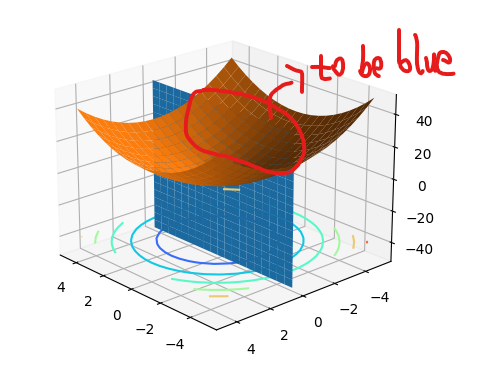# Show the part of a plane before a part of a curved surface

hello there，I want to show the blue part of the plane rather than the orange one. In other words, I want the blue part that is over the orange to be shown in the screen. How can I do that?My code is below:

``````import matplotlib.pyplot as plt
import numpy as np

fig = plt.figure()
axes = plt.axes(projection='3d')

w1 = np.arange(-5,5,0.5)
w2 = np.arange(-5,5,0.5)
w1,w2 = np.meshgrid(w1,w2)
L = w1**2+w2**2  # 函数定义
L3 = 10*w1.T

curve2 = axes.plot_surface(w1,np.zeros_like(w1),L3)
curve2.set_zorder(3)

curve1 = axes.plot_surface(w1,w2,L)  # 画函数
curve1.set_zorder(2)

axes.contour(w1,w2,L, zdim='z',offset=-40,cmap='rainbow')  # 画等高线
``````

Matplotlib does not have true 3D rendering. So each artist is drawn separately and in ignorance of the others. In this case you could try and divide the surface.